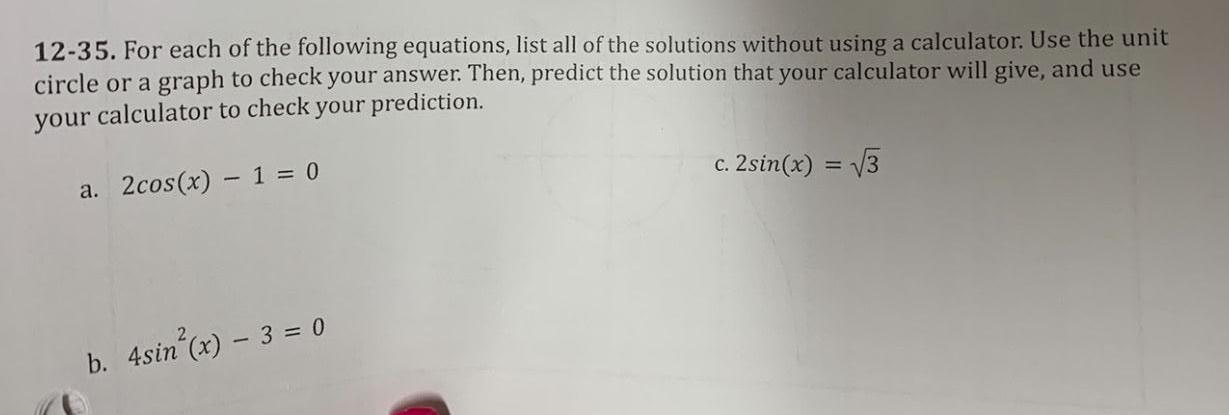Question:

# 12-35. For each of the following equations, list all of the

Last updated: 8/18/202212-35. For each of the following equations, list all of the solutions without using a calculator. Use the unit circle or a graph to check your answer. Then, predict the solution that your calculator will give, and use your calculator to check your prediction. a. 2cos(x) - 1 = 0 b. 4sin²(x) - 3 = 0 c. 2sin(x) = √3